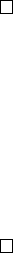5Fourier transform

II Probability and Measure5.2 Convolutions
To actually do something useful about the Fourier transforms, we need to talk
Definition
(Convolution of random variables)
.
Let
µ, ν
be probability measures.
Their convolution
µ ν
is the law of
X
+
Y
, where
X
has law
µ
and
Y
has law
ν, and X, Y are independent. Explicitly, we have
µ ν(A) = P[X + Y A]
=
ZZ
1
A
(x + y) µ(dx) ν(dy)
Let’s suppose that
µ
has a density function
f
with respect to the Lebesgue
measure. Then we have
µ ν(A) =
ZZ
1
A
(x + y)f(x) dx ν(dy)
=
ZZ
1
A
(x)f(x y) dx ν(dy)
=
Z
1
A
(x)
Z
f(x y) ν(dy)
dx.
So we know that µ ν has law
Z
f(x y) ν(dy).
This thing has a name.
Definition
(Convolution of function with measure)
.
Let
f L
p
and
ν
a
probability measure. Then the convolution of f with µ is
f ν(x) =
Z
f(x y) ν(dy) L
p
.
Note that we do have to treat the two cases of convolutions separately, since
a measure need not have a density, and a function need not specify a probability
measure (it may not integrate to 1).
We check that it is indeed in L
p
. Since ν is a probability measure, Jensen’s
inequality says we have
kf νk
p
p
=
Z
Z
|f(x y)|ν(dy)
p
dx
ZZ
|f(x y)|
p
ν(dy) dx
=
ZZ
|f(x y)|
p
dx ν(dy)
= kfk
p
p
< .
In fact, from this computation, we see that
Proposition. For any f L
p
and ν a probability measure, we have
kf νk
p
kf k
p
.
The interesting thing happens when we try to take the Fourier transform of
a convolution.
Proposition.
[
f ν(u) =
ˆ
f(u)ˆν(u).
Proof. We have
[
f ν(u) =
Z
Z
f(x y)ν(dy)
e
i(u,x)
dx
=
ZZ
f(x y)e
i(u,x)
dx ν(dy)
=
Z
Z
f(x y)e
i(u,xy)
d(x y)
e
i(u,y)
µ(dy)
=
Z
Z
f(x)e
i(u,x)
d(x)
e
i(u,y)
µ(dy)
=
Z
ˆ
f(u)e
i(u,x)
µ(dy)
=
ˆ
f(u)
Z
e
i(u,x)
µ(dy)
=
ˆ
f(u)ˆν(u).
In the context of random variables, we have a similar result:
Proposition.
Let
µ, ν
be probability measures, and
X, Y
be independent vari-
ables with laws µ, ν respectively. Then
[
µ ν(u) = ˆµ(u)ˆν(u).
Proof. We have
[
µ ν(u) = E[e
i(u,X+Y )
] = E[e
i(u,X)
]E[e
i(u,Y )
] = ˆµ(u)ˆν(u).